# Mathematics on the fingers GIFCA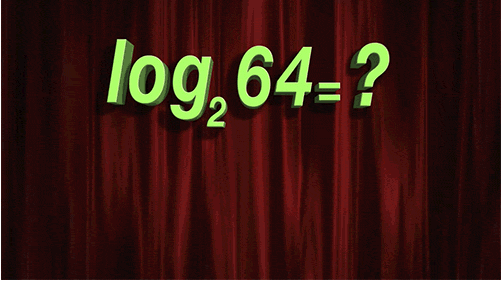Mathematics (ancient Greek <ancient Greek learning, science..) - The science of structures, procedures and relationships historically established on the basis of counting operations, measuring and describing the shapes of objects. Mathematical objects are created by the idealization of real property or other mathematical objects and record these properties in a formal language. Mathematics is not a science, but it is widely used in them as to their exact wording of the content and to obtain new results.

Mathematics - basic science, providing a (common) language means other sciences; thus it reveals its structural relationship and helps finding the most general laws of nature.

Animated Math is not dry charts and figures, which are sometimes impossible to understand. This is understandable, visual animated gifs fundamental laws of mathematics. This is looked at SIFCO and more, from school, it becomes clear. Watch interesting even to those who did not understand anything;)

## How to draw an ellipse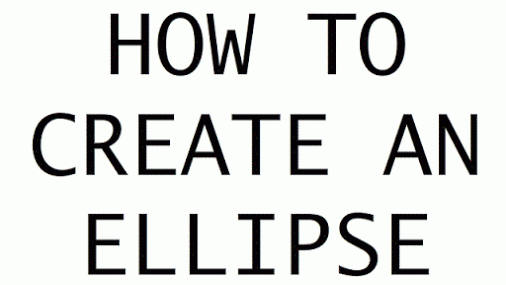Ellipse - a closed curve in the plane, which can be obtained as the intersection of the plane and a circular cylinder or as an orthogonal projection onto the plane of the circle.

## How does Pascal's triangle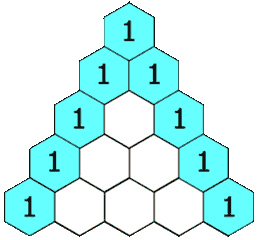Pascal's Triangle - on the top and sides of the unit, each number is the sum of the numbers placed on them.

## How quickly multiply binomialBean or binomial - a special case of a polynomial (polynomial), consisting of two terms of monomials (monomials).

## How to understand logarithms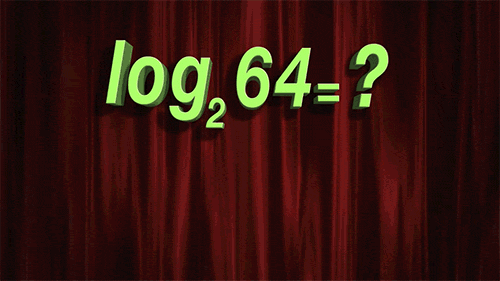What logarithms?

## How not to get confused by conducting matrix transposition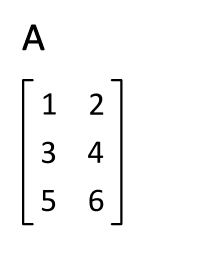Carrying out the matrix transposition.

## Intuitively, that "Pythagorean pants in all directions are equal"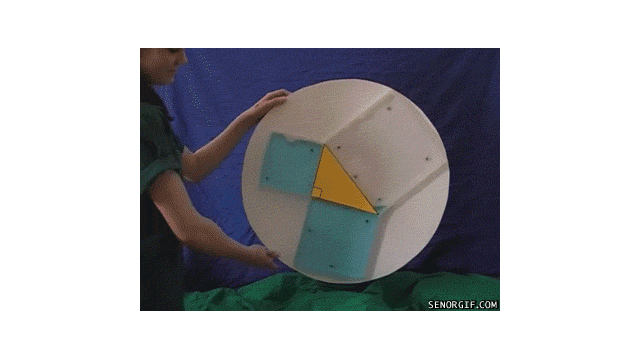a vivid example of the rule of "Pythagorean pants on all sides are equal."

## Why is the amount of external corners of the polygon is always equal to 360 degrees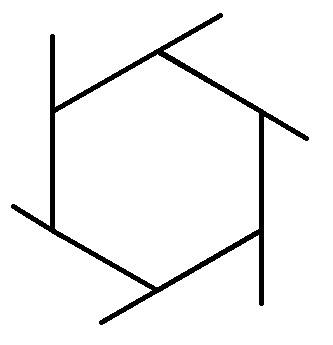Intuitively, the amount of external corners of the polygon.

## How to actually look like the number "Pi"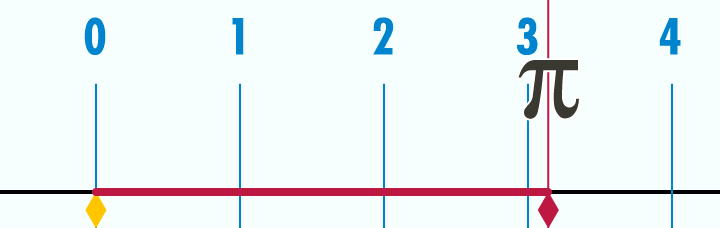As the number of "Pi" looks really? There you are.

## If the arc length is equal to the length of its radius, the resulting angle is equal to one radianIf the arc length is equal to the length of its radius, the resulting angle is equal to one radian.

## Visualization sinus (red) and cosine (blue) corner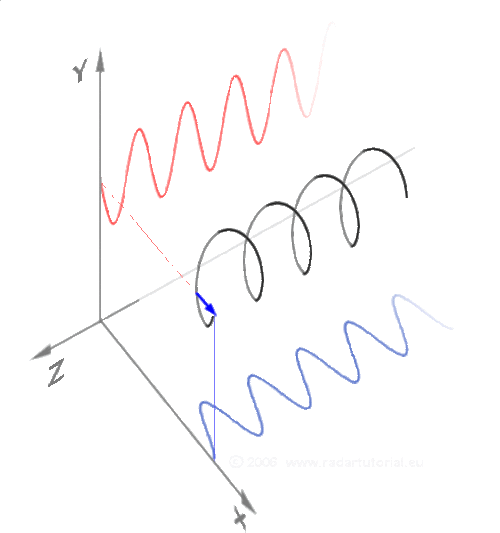Visualization sinus (red) and cosine (blue) corner. There you are.

## The same, but even easier## Same triangles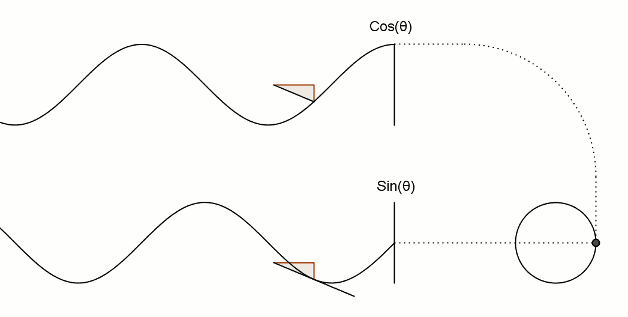## And a little trigonometry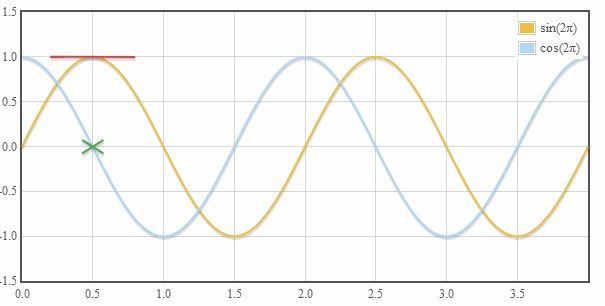## The curves of tan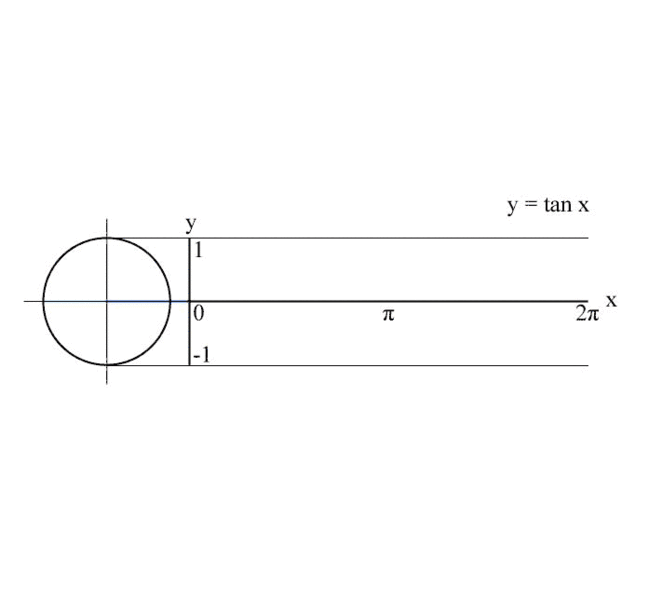The curves of tan? There you are.

## If you expand the picture gets even clearer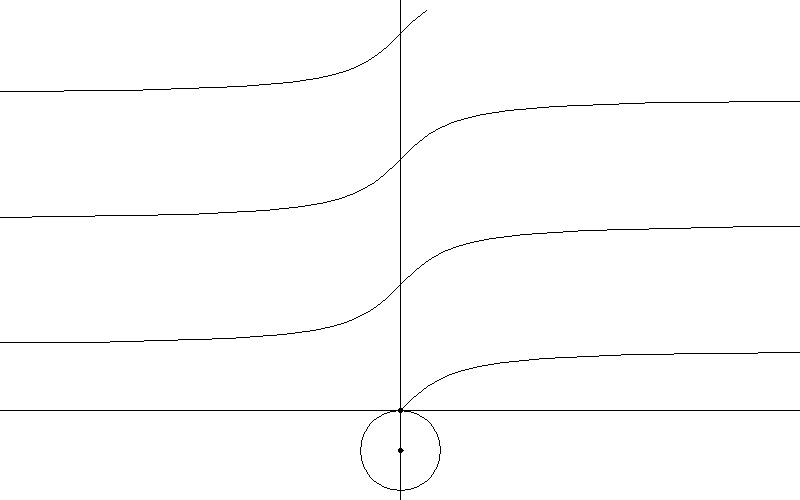## How do I transfer function from the Cartesian to polar coordinate system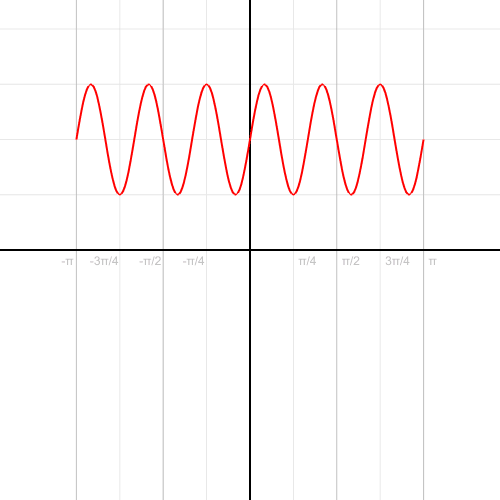How do I transfer function from the Cartesian to the polar coordinate system? There you are.

## How to draw a parabola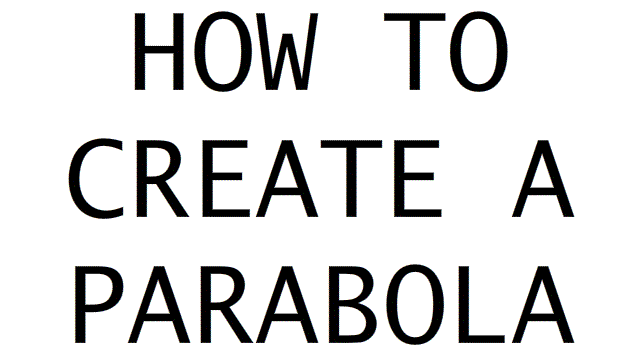How to draw a parabola? There you are.

## Riemann sum - the approximate area under the curve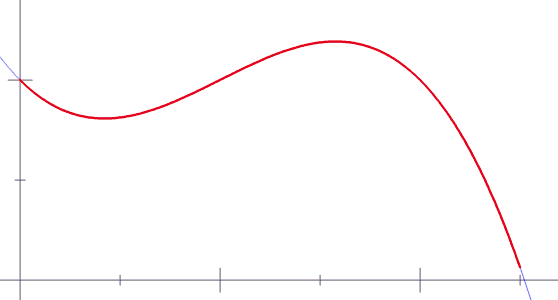The sum of the Riemann - approximate area under the curve. There you are.

## How to draw a hyperbolaPraivlny way to draw a hyperbole.

## If we translate this in 3D, will hyperboloid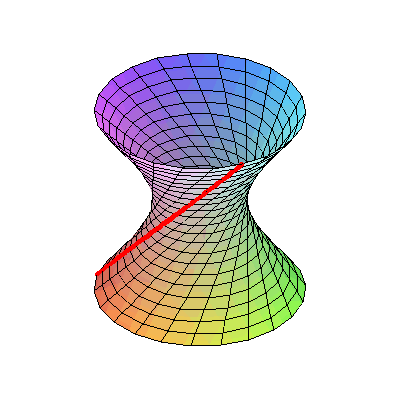Schematic representation of the hyperboloid. That's all - straight lines, though hard to believe!

## Here is a hyperboloid in real life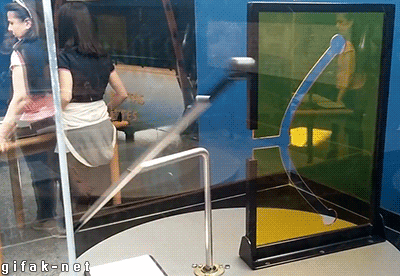How does the hyperboloid in real life!

## Mathematical animated graphics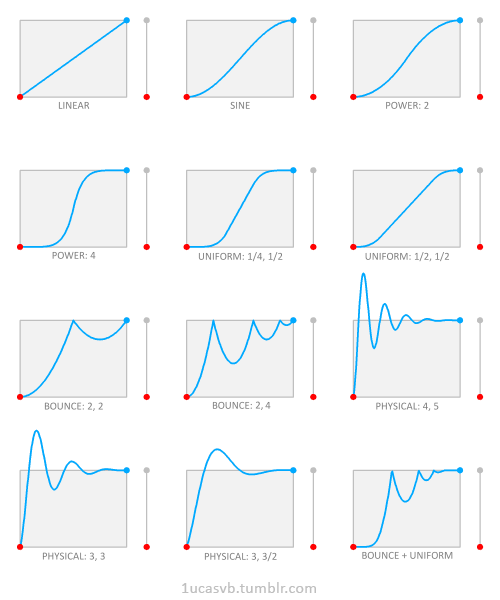Various physical and mathematical charts.